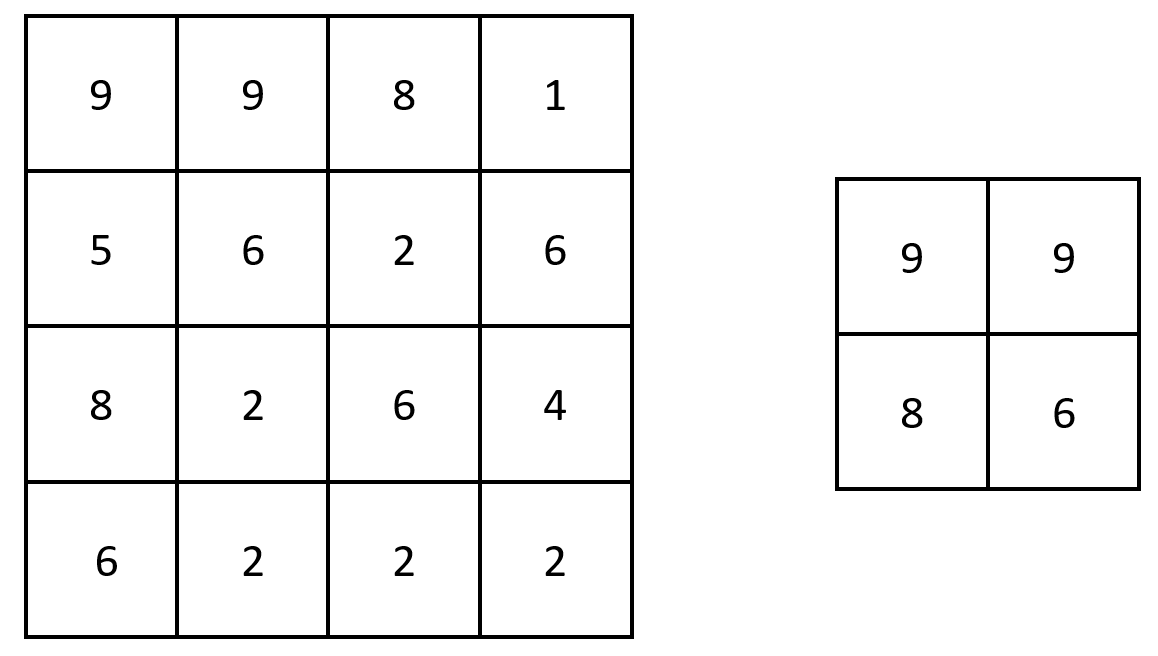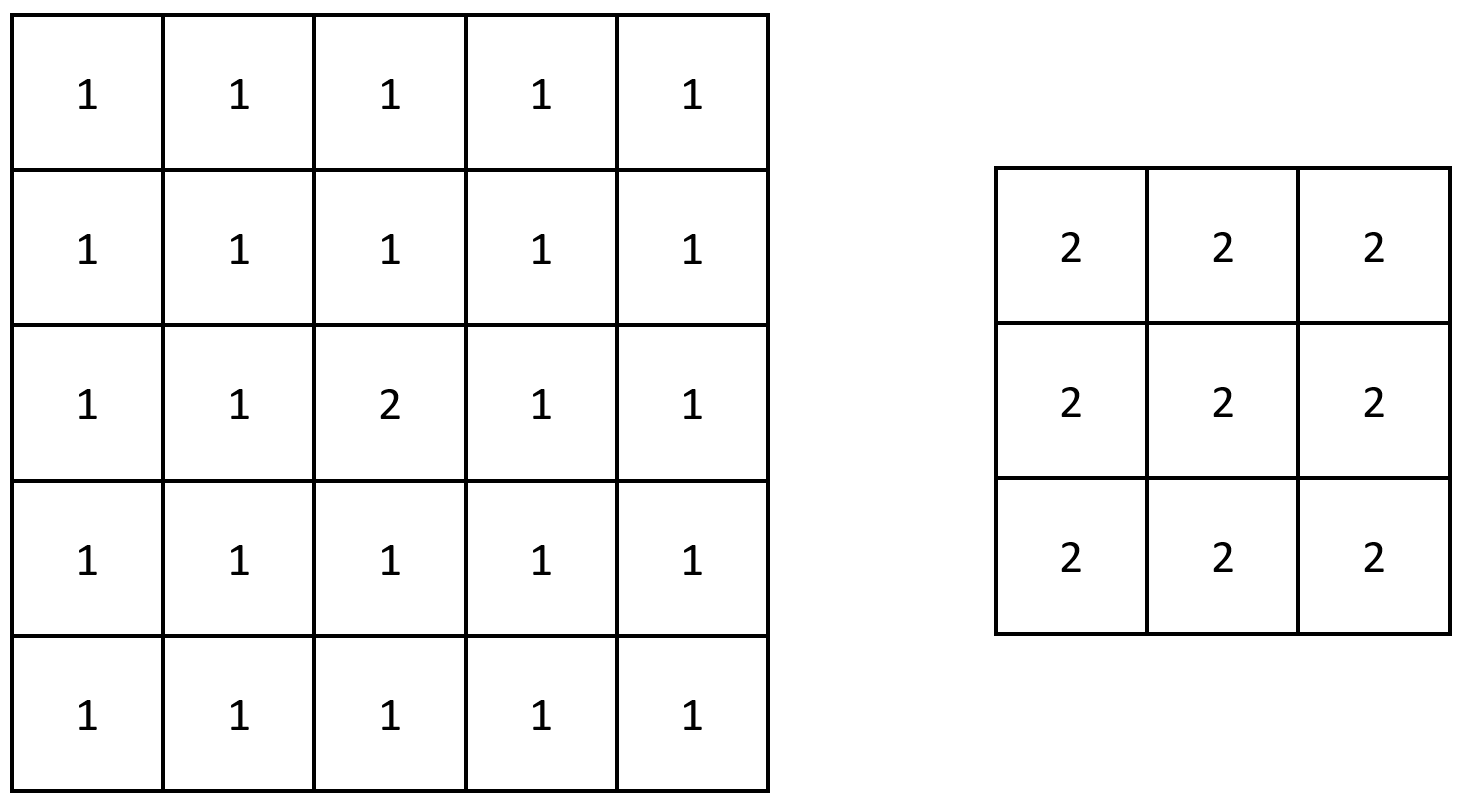2373. Largest Local Values in a Matrix
Easy
548
59

You are given an `n x n` integer matrix `grid`.

Generate an integer matrix `maxLocal` of size `(n - 2) x (n - 2)` such that:

• `maxLocal[i][j]` is equal to the largest value of the `3 x 3` matrix in `grid` centered around row `i + 1` and column `j + 1`.

In other words, we want to find the largest value in every contiguous `3 x 3` matrix in `grid`.

Return the generated matrix.

Example 1:```Input: grid = [[9,9,8,1],[5,6,2,6],[8,2,6,4],[6,2,2,2]]
Output: [[9,9],[8,6]]
Explanation: The diagram above shows the original matrix and the generated matrix.
Notice that each value in the generated matrix corresponds to the largest value of a contiguous 3 x 3 matrix in grid.```

Example 2:```Input: grid = [[1,1,1,1,1],[1,1,1,1,1],[1,1,2,1,1],[1,1,1,1,1],[1,1,1,1,1]]
Output: [[2,2,2],[2,2,2],[2,2,2]]
Explanation: Notice that the 2 is contained within every contiguous 3 x 3 matrix in grid.
```

Constraints:

• `n == grid.length == grid[i].length`
• `3 <= n <= 100`
• `1 <= grid[i][j] <= 100`
Accepted
37.3K
Submissions
44.8K
Acceptance Rate
83.2%

Seen this question in a real interview before?
1/4
Yes
No

Discussion (0)

Related Topics